# Ohio EOCE Integrated Math I: Functions Overview Chapter Exam

Exam Instructions:

Choose your answers to the questions and click 'Next' to see the next set of questions. You can skip questions if you would like and come back to them later with the yellow "Go To First Skipped Question" button. When you have completed the practice exam, a green submit button will appear. Click it to see your results. Good luck!

### Page 1

#### Question 1 1. What is the domain of the function below?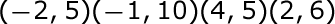#### Question 2 2. Use the graph to find f(f(2)).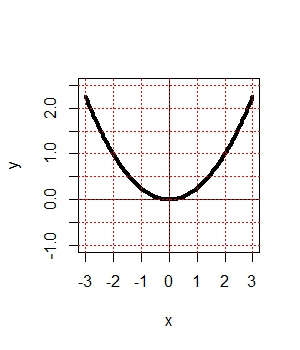#### Question 5 5. What is the inverse function of f(x) = 3(x - 2)3?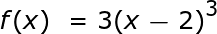### Page 2

#### Question 6 6. The revenue of a company is represented by R(x) and their costs are represented by C(x). What is the profit function R(x) - C(x)?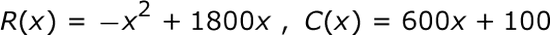### Page 4

#### Question 16 16. Remembering function notation, we want x to be w. Therefore, if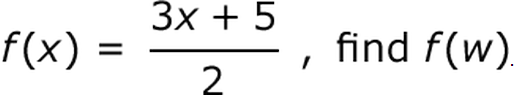#### Question 17 17. Which interval(s) offers a solution?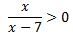#### Question 19 19. Evaluate f(f(x)). [Yes, it says f(f(x)]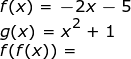### Page 5

#### Question 24 24. Find the domain of f(x)/g(x), if: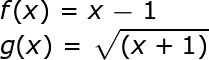### Page 6

#### Question 26 26. Using the function below, evaluate g(7).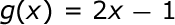#### Question 27 27. Using the graph, find f(f(-2)).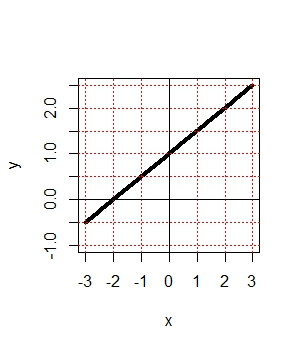#### Ohio EOCE Integrated Math I: Functions Overview Chapter Exam Instructions

Choose your answers to the questions and click 'Next' to see the next set of questions. You can skip questions if you would like and come back to them later with the yellow "Go To First Skipped Question" button. When you have completed the practice exam, a green submit button will appear. Click it to see your results. Good luck!

Support